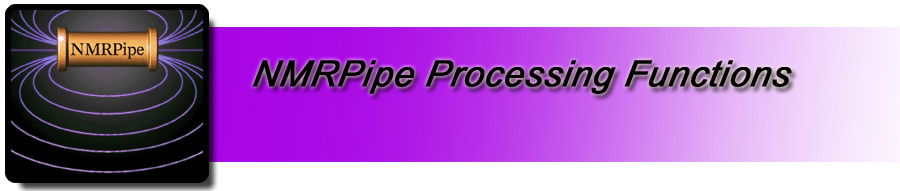NMRPipe Processing Functions

This particular reference page lists the specific processing functions that can be used in an nmrPipe processing pipeline (via nmrPipe -fn ...), for example "FT", the Fourier Transform function.

Like FT, many of these functions frequently in typical processing schemes. Some functions and options, however, may rarely be used, were added only for development purposes, or may be superseded by other functions. In an attempt to indicate which functions and options are used most frequently, this reference list includes an instance count. In the current version of this reference list, the instance count tallies how often NMRPipe's developer Frank Delaglio has used a particular function or option in a script during both routine use and research and development of the software. Note that this scheme will underestimate the uses of functions such as SAVE which are primarily used interactively, and not within scripts.Function Instances Description Common Arguments (Instances) ADD 0 Add a Constant APOD 11 Apply the Selected Window (Apodization) `-inv (7)` `-hdr (7)` `-qName (4)` `-q1 (4)` `-q2 (4)` `-q3 (4)` BASE 9 Linear Baseline Corrections `-first (8)` `-last (8)` `-nw (8)` `-nl (6)` CBF 20 Subtract Constant Baseline COADD 31 Linear Combination of Data Vectors `-cList (30)` `-axis (27)` `-time (25)` CS 48 Circular Shift `-rs (37)` `-sw (37)` `-neg (27)` `-ls (10)` `-1ppm (5)` `-inv (1)` DX 0 Derivative EM 35 Exponential Multiply Window `-lb (34)` `-c (18)` EXT 487 Extract Region `-sw (455)` `-xn (317)` `-x1 (306)` `-left (157)` `-yn (7)` `-y1 (6)` FSH 2 Frequency Shift via Fourier Transform `-ls (1)` `-sw (1)` FT 1597 Complex Fourier Transform `-inv (178)` `-auto (111)` `-neg (53)` `-alt (25)` `-bruk (17)` `-real (11)` GM 54 Lorentz-to-Gauss Window `-g1 (53)` `-g2 (53)` `-c (53)` `-g3 (5)` GMB 0 Gaussian Window HA 0 Hadamard Transform HT 155 Reconstruct Imaginary Data Via Hilbert Transform `-auto (90)` `-ps90-180 (4)` IMG 5 Image Processing Functions `-median (3)` `-dx (2)` `-dy (2)` `-kern (1)` `-min (1)` `-thresh (1)` INTEG 0 Integral JMOD 0 Damped J-Modulation LP 198 Linear Prediction `-ord (148)` `-fb (93)` `-pred (74)` `-xn (46)` `-ps0-0 (28)` `-ps90-180 (18)` LS 0 Left Shift MAC 187 M-language MACRO Processing `-macro (186)` `-all (67)` `-var (25)` `-str (14)` `-reg (9)` `-dif (6)` MC 29 Modulus (Magnitude) Calculation for Complex Data `-p0 (4)` `-p1 (4)` MED 2 Baseline Correction via Local Median `-nw (1)` MEM 50 ND Maximum Entropy Reconstruction `-sigma (49)` `-report (46)` `-ndim (42)` `-alpha (21)` `-zero (18)` `-xconv (17)` MIR 2 Form Mirror Image ML 22 Maximum Likelihood Frequency Map `-xfSize (12)` `-ndim (11)` `-yfSize (10)` `-xlw (8)` `-sign (7)` `-report (5)` MULT 92 Multiply by a Constant `-xn (69)` `-c (63)` `-hdr (24)` `-inv (6)` `-r (4)` `-i (2)` NULL 7 Null Function; Leave Data Unchanged POLY 169 Polynomial Time-Domain Solvent Subtraction `-time (169)` POLY 562 Polynomial Frequency-Domain Baseline Correction `-auto (390)` `-ord (134)` `-xn (20)` `-x1 (20)` `-window (3)` `-nw (3)` PS 1333 Phase Correction `-p0 (1176)` `-p1 (1167)` `-hdr (150)` `-inv (89)` `-ls (2)` `-rs (2)` QART 6 Scale Quad Artifacts `-a (5)` `-f (5)` QMIX 5 General Linear Combination of Data Vectors `-ic (5)` `-oc (5)` `-cList (5)` `-time (5)` `-0,1 (1)` REV 17 Reverse Data `-sw (2)` RFT 0 Real Fourier Transform RS 22 Right Shift `-rs (21)` `-sw (11)` SAVE 0 Save Current 1D Data Vector SET 43 Set Data to Constant `-r (25)` `-c (15)` `-x1 (9)` `-xn (8)` SHUF 15 Shuffle Data (Exchange Reals and Imaginaries, etc) `-exlr (7)` `-ri2c (2)` `-bswap (2)` `-swap (1)` `-c2ri (1)` `-r2i (1)` SIGN 11 Sign Manipulation (Negate, etc) `-alt (7)` `-i (3)` SMO 3 Smooth Data via Convolution Filter SOL 208 Solvent Filter `-head (16)` `-mir (16)` `-fl (2)` SP 1357 Adjustable Sine Bell Window `-off (1204)` `-end (1160)` `-pow (1148)` `-c (1143)` `-inv (117)` `-hdr (117)` TM 0 Trapezoid Window TP 892 2D Transpose XY->YX (YTP) `-hyper (47)` `-nohdr (7)` `-auto (2)` TRI 2 Triangle Window `-loc (1)` ZD 0 Zero Diagonal Region ZF 1580 Zero Fill `-auto (941)` `-size (284)` `-zf (169)` `-inv (122)` `-mid (11)` `-pad (3)` ZTP 35 3D Transpose XYZ->ZYX

[
Home ] [ NIH ] [ NIDDK ] [ Disclaimer ] [ Copyright ]
last updated: Jul 24, 2011 / Webmaster